# ISEE Upper Level Quantitative : How to find if two triangles are similar

## Example Questions

### Example Question #23 : Plane Geometry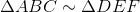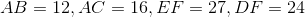Which is the greater quantity?

(a)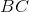(b)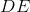(b) is greater

It is impossible to tell from the information given

(a) is greater

(a) and (b) are equal

(a) and (b) are equal

Explanation:, so by definition, the sides are in proportion.

(a)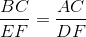Substitute and solve for: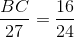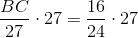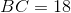(b)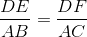Substitute and solve for: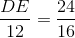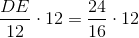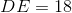The two are equal.

### Example Question #24 : Plane Geometry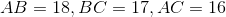Which is the greater quantity?

(a)(b)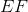(a) and (b) are equal.

It is impossible to tell from the information given.

(b) is greater.

(a) is greater.

(a) is greater.

Explanation:, so by definition, the sides are in proportion. Therefore,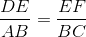.

Substitute: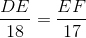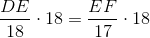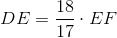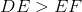, so (a) is greater.